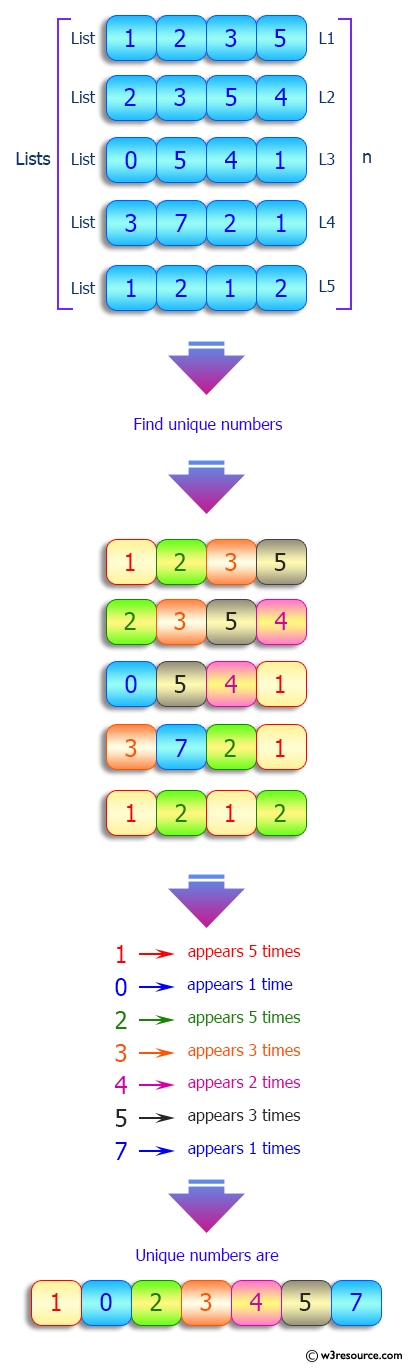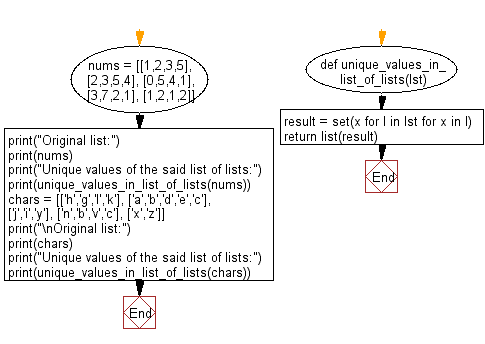﻿ Python: Unique values in a given list of lists - w3resource# Python: Unique values in a given list of lists

## Python List: Exercise - 183 with Solution

Write a Python program to get the unique values in a given list of lists.

Sample Solution:

Python Code:

``````def unique_values_in_list_of_lists(lst):
result = set(x for l in lst for x in l)
return list(result)
nums = [[1,2,3,5], [2,3,5,4], [0,5,4,1], [3,7,2,1], [1,2,1,2]]
print("Original list:")
print(nums)
print("Unique values of the said list of lists:")
print(unique_values_in_list_of_lists(nums))
chars = [['h','g','l','k'], ['a','b','d','e','c'], ['j','i','y'], ['n','b','v','c'], ['x','z']]
print("\nOriginal list:")
print(chars)
print("Unique values of the said list of lists:")
print(unique_values_in_list_of_lists(chars))
```
```

Sample Output:

```Original list:
[[1, 2, 3, 5], [2, 3, 5, 4], [0, 5, 4, 1], [3, 7, 2, 1], [1, 2, 1, 2]]
Unique values of the said list of lists:
[0, 1, 2, 3, 4, 5, 7]

Original list:
[['h', 'g', 'l', 'k'], ['a', 'b', 'd', 'e', 'c'], ['j', 'i', 'y'], ['n', 'b', 'v', 'c'], ['x', 'z']]
Unique values of the said list of lists:
['e', 'd', 'c', 'b', 'x', 'k', 'n', 'h', 'g', 'j', 'i', 'a', 'l', 'y', 'v', 'z']
```

Pictorial Presentation:Flowchart:## Visualize Python code execution:

The following tool visualize what the computer is doing step-by-step as it executes the said program:

Python Code Editor:

Have another way to solve this solution? Contribute your code (and comments) through Disqus.

What is the difficulty level of this exercise?

Test your Python skills with w3resource's quiz

﻿

## Python: Tips of the Day

Floor Division:

When we speak of division we normally mean (/) float division operator, this will give a precise result in float format with decimals.

For a rounded integer result there is (//) floor division operator in Python. Floor division will only give integer results that are round numbers.

```print(1000 // 300)
print(1000 / 300)```

Output:

```3
3.3333333333333335```